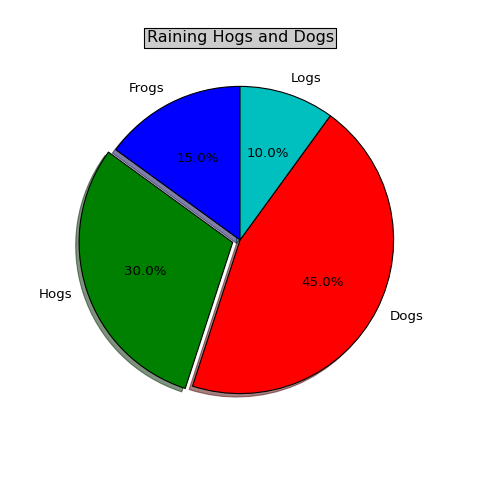pylab_examples example code: pie_demo.py¶"""
Make a pie chart - see
http://matplotlib.sf.net/matplotlib.pylab.html#-pie for the docstring.

This example shows a basic pie chart with labels optional features,
like autolabeling the percentage, offsetting a slice with "explode",

"""
from pylab import *

# make a square figure and axes
figure(1, figsize=(6,6))
ax = axes([0.1, 0.1, 0.8, 0.8])

# The slices will be ordered and plotted counter-clockwise.
labels = 'Frogs', 'Hogs', 'Dogs', 'Logs'
fracs = [15, 30, 45, 10]
explode=(0, 0.05, 0, 0)

pie(fracs, explode=explode, labels=labels,